# Geometry Shaalaa.com Model Set 4 2019-2020 SSC (Marathi Semi-English) Class 10th Board Exam Question Paper Solution

Geometry [Shaalaa.com Model Set 4]
Date: March 2020
Duration: 2h

1
1.A | MCQs
1.A.i

In the given figure, seg XY || seg BC, then which of the following statements is true?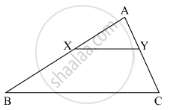$\frac{AB}{AC} = \frac{AX}{AY}$

$\frac{AX}{XB} = \frac{AY}{AC}$

$\frac{AX}{YC} = \frac{AY}{XB}$

$\frac{AB}{YC} = \frac{AC}{XB}$

Concept: Similarity of Triangles
Chapter:  Similarity
1.A.ii

Select the correct alternative for the following question.

(3) If ∆ABC ~ ∆PQR and (AB)/(PQ) = 7/5, then ...............

∆ABC is bigger.

∆PQR is bigger.

Both triangles will be equal.

Can not be decided.

Concept: Construction of Tangent to the Circle from the Point on the Circle
Chapter:  Geometric Constructions
1.A.iii

Some question and their alternative answer are given. Select the correct alternative.

Altitude on the hypotenuse of a right angled triangle divides it in two parts of lengths 4 cm and 9 cm. Find the length of the altitude.

9 cm

4 cm

6 cm

$2\sqrt{6}$ cm

Concept: Apollonius Theorem
Chapter:  Pythagoras Theorem
1.A.iv

Choose the correct alternative.

∠ACB is inscribed in arc ACB of a circle with centre O. If ∠ACB = 65°, find m(arc ACB).

65°

130°

295°

230°

Concept: Inscribed Angle
Chapter:  Circle
1.B
1.B.i

In the given figure, BC ⊥ AB, AD ⊥ AB, BC = 4, AD = 8, then find $\frac{A\left( ∆ ABC \right)}{A\left( ∆ ADB \right)} .$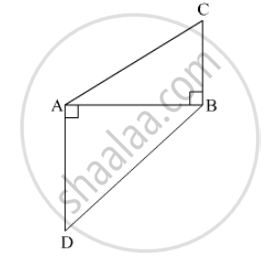Concept: Similar Triangles
Chapter:  Similarity
1.B.ii

∆AMT ~ ∆AHE. In ∆AMT, AM = 6.3 cm, ∠TAM = 50°, AT = 5.6 cm.  $\frac{AM}{AH} = \frac{7}{5} .$Construct ∆AHE.

Concept: Division of a Line Segment
Chapter:  Co-ordinate Geometry  Geometric Constructions
1.B.iii

Determine whether the following point is collinear.

D(–2, –3), E(1, 0), F(2, 1)

Concept: Slope of a Line
Chapter:  Co-ordinate Geometry
1.B.iv

Find the surface area and the volume of a beach ball shown in the figure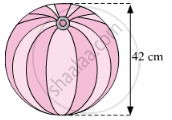Concept: Surface Area of a Combination of Solids
Chapter:  Mensuration
2
2.A | Solve any 2 of the following
2.A.i

Are the triangles in the given figure similar? If yes, by which test ?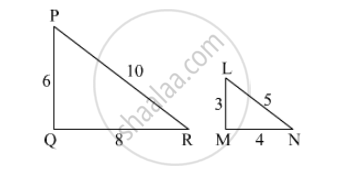Concept: Similarity of Triangles
Chapter:  Similarity
2.A.ii

In ∆ABC, point M is the midpoint of side BC.
If, AB+ AC= 290 cm2, AM = 8 cm, find BC.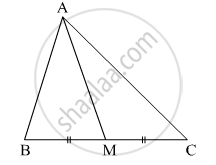Concept: Apollonius Theorem
Chapter:  Pythagoras Theorem
2.A.iii

In the given figure, O is the centre of the ci
rcle. Seg AB, seg AC are tangent segments. Radius of the circle is r and l(AB) = r , Prove that, ▢ABOC is a square.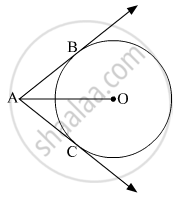Concept: Tangent Segment Theorem
Chapter:  Circle
2.B | Solve any 4 of the following
2.B.i

∆ABC ~ ∆LBN. In ∆ABC, AB = 5.1cm, ∠B = 40°, BC = 4.8 cm,$\frac{AC}{LN} = \frac{4}{7}$. Construct ∆ABC and ∆LBN.

Concept: Division of a Line Segment
Chapter:  Co-ordinate Geometry  Geometric Constructions
2.B.ii

From the top of the light house, an observer looks at a ship and finds the angle of depression to be 30°. If the height of the light-house is 100 meters, then find how far the ship is from the light-house.

Concept: Heights and Distances
Chapter:  Trigonometry
2.B.iii

If $\sin\theta = \frac{7}{25}$, find the values of cosθ and tan​θ.

Concept: Application of Trigonometry
Chapter:  Trigonometry
2.B.iv

In the given figure, O is the centre of the circle and B is a point of contact. seg OE ⊥ seg AD, AB = 12, AC = 8, find
(1) AD (2) DC (3) DE.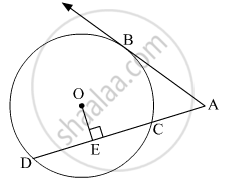Concept: Tangent Segment Theorem
Chapter:  Circle
2.B.v

In the given figure, a cylindrical wrapper of flat tablets is shown. The radius of a tablet is 7 mm and its thickness is 5 mm. How many such tablets are wrapped in the wrapper?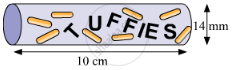Concept: Surface Area of a Combination of Solids
Chapter:  Mensuration
3
3.A | Solve any 1 of the following
3.A.i

If ∆ABC ~ ∆PQR, A (∆ABC) = 80, A (∆PQR) = 125, then fill in the blanks. $\frac{A\left( ∆ ABC \right)}{A\left( ∆ . . . . \right)} = \frac{80}{125} \therefore \frac{AB}{PQ} = \frac{......}{......}$

Concept: Areas of Two Similar Triangles
Chapter:  Similarity
3.A.ii

In the given figure, M is the centre of the circle and seg KL is a tangent segment.
If MK = 12, KL = $6\sqrt{3}$ then find –
(2) Measures of ∠K and ∠M.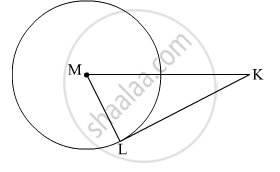Concept: Tangent Segment Theorem
Chapter:  Circle
3.B | Solve any 2 of the following
3.B.i

In the given figure, the vertices of square DEFG are on the sides of ∆ABC. ∠A = 90°. Then prove that DE2 = BD × EC (Hint: Show that ∆GBD is similar to ∆CFE. Use GD = FE = DE.)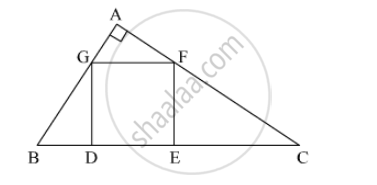Concept: Property of three parallel lines and their transversals
Chapter:  Similarity
3.B.ii

Draw a circle with centre P. Draw an arc AB of 100° measure. Draw tangents to the circle at point A and point B.

Concept: Construction of Tangents to a Circle
Chapter:  Geometric Constructions
3.B.iii

Find the coordinates of circumcentre and radius of circumcircle of ∆ABC if A(7, 1), B(3, 5) and C(2, 0) are given.

Concept: Concepts of Coordinate Geometry
Chapter:  Co-ordinate Geometry
3.B.iv

The line segment AB is divided into five congruent parts at P, Q, R and S such that A–P–Q–R–S–B. If point Q(12, 14) and S(4, 18) are given find the coordinates of A, P, R, B.

Concept: Division of a Line Segment
Chapter:  Co-ordinate Geometry  Geometric Constructions
4 | Solve any 2 of the following
4.A

In a trapezium ABCD, seg AB || seg DC seg BD ⊥ seg AD, seg AC ⊥ seg BC, If AD = 15, BC = 15 and AB = 25. Find A(▢ABCD)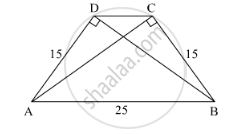Concept: Pythagoras Theorem
Chapter:  Pythagoras Theorem
4.B

In the given figure, a ∆ABC is drawn to circumscribe a circle of radius 4 cm such that the segments BD and DC are of lengths 8 cm and 6 cm respectively. Find the lengths of sides AB and AC, when area of ∆ABC is 84 cm2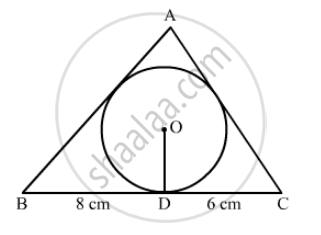Concept: Concept of Circle - Centre, Radius, Diameter, Arc, Sector, Chord, Segment, Semicircle, Circumference, Interior and Exterior.
Chapter:  Circle
4.C

The difference between outer and inner curved surface areas of a hollow right circular cylinder 14cm long is 88cm2. If the volume of metal used in making cylinder is 176cm3.find the outer and inner diameters of the cylinder____?

Concept: Volume of a Combination of Solids
Chapter:  Mensuration
5 | Solve any 1 of the following
5.A

Prove that:

$\frac{\tan\theta}{\sec\theta - 1} = \frac{\tan\theta + \sec\theta + 1}{\tan\theta + \sec\theta - 1}$
Concept: Application of Trigonometry
Chapter:  Trigonometry
5.B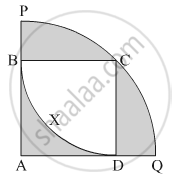In the given figure, square ABCD is inscribed in the sector A - PCQ. The radius of sector C - BXD is 20 cm. Complete the following activity to find the area of shaded region

Concept: Surface Area of a Combination of Solids
Chapter:  Mensuration

#### Request Question Paper

If you dont find a question paper, kindly write to us

View All Requests

#### Submit Question Paper

Help us maintain new question papers on Shaalaa.com, so we can continue to help students

only jpg, png and pdf files

## Maharashtra State Board previous year question papers Class 10th Board Exam Geometry with solutions 2019 - 2020

Maharashtra State Board Class 10th Board Exam Geometry question paper solution is key to score more marks in final exams. Students who have used our past year paper solution have significantly improved in speed and boosted their confidence to solve any question in the examination. Our Maharashtra State Board Class 10th Board Exam Geometry question paper 2020 serve as a catalyst to prepare for your Geometry board examination.
Previous year Question paper for Maharashtra State Board Class 10th Board Exam Geometry-2020 is solved by experts. Solved question papers gives you the chance to check yourself after your mock test.
By referring the question paper Solutions for Geometry, you can scale your preparation level and work on your weak areas. It will also help the candidates in developing the time-management skills. Practice makes perfect, and there is no better way to practice than to attempt previous year question paper solutions of Maharashtra State Board Class 10th Board Exam.

How Maharashtra State Board Class 10th Board Exam Question Paper solutions Help Students ?
• Question paper solutions for Geometry will helps students to prepare for exam.
• Question paper with answer will boost students confidence in exam time and also give you an idea About the important questions and topics to be prepared for the board exam.
• For finding solution of question papers no need to refer so multiple sources like textbook or guides.